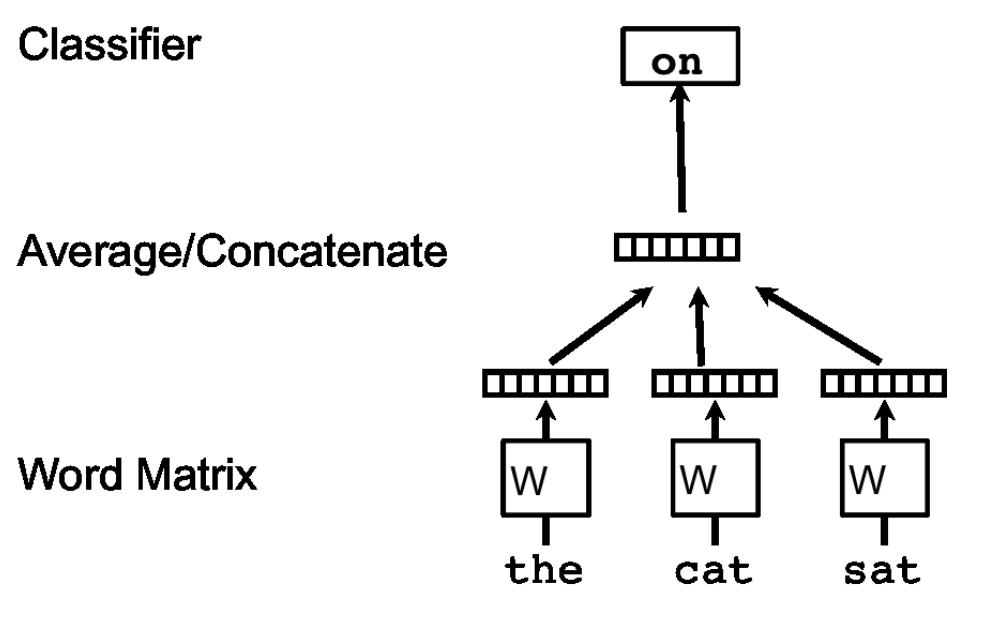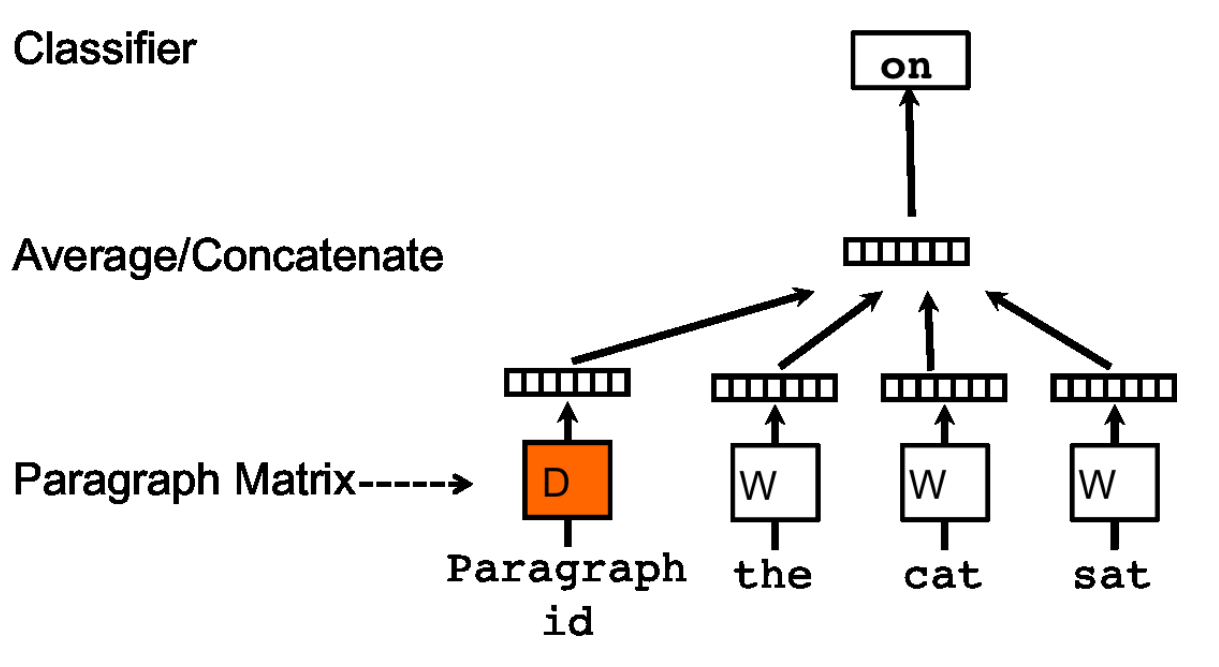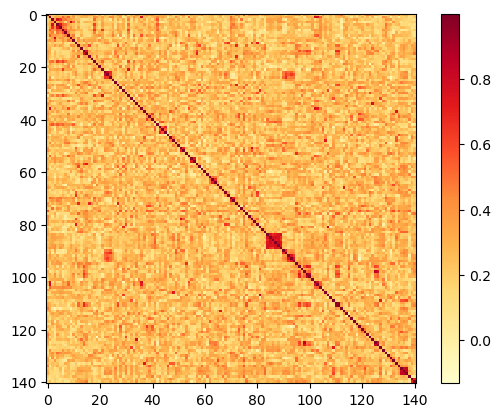# Similar posts recommendation with Doc2Vec - Part I

Marton Trencseni - Sat 03 December 2022 - machine-learning

## Introduction

One of the things I learned at Facebook is the power of recommendations. Examples are People You May Know (PYMK), Groups You May Like (GYML) and Pages You May Like (PYML). These products (little boxes) are all over Facebook and are one of the core drivers of engagement. For example, here's a snapshot of my current People You May Know recommendation (check yours here):Inspired by these, I am planning to add Articles You May Like recommendations to Bytepawn (this blog), based on the semantic similarity of my blog posts. I use the Doc2Vec neural network architecture from the gensim library to compute the similarity between my blog posts, and return the top 3 recommendations for each page. The code is up on Github.

## Extracting post contents

The first step is easy: for each post, I want to get an id and the contents of the post. Bytepawn is based on Pelican, a static blog generator, which means each post is a Markdown file, structured like:

Title: Similar posts recommendation with Doc2Vec - Part I
Date: 2022-12-03
Modified: 2022-12-03
Category: machine-learning
Tags: similarity, python, gensim, word2vec, doc2vec, pyml
Slug: similar-posts-recommendation-with-doc2vec
Authors: Marton Trencseni
Summary: One of the things I learned at Facebook is the power of recommendations...
Image: /images/doc2vec_similarity_matrix.png

Introduction
------------
One of the things I learned at Facebook is the power of recommendations.
...


Parsing these files is trivial, using the slugs as ids:

import os

def build_post_struct(lines):
slug = next(line[len('slug:'):].strip() for line in lines[:10] if line.lower().startswith('slug:'))
return {'slug': slug, 'contents': '\n'.join(lines[10:]).lower()}

BLOG_DIR = "d:/code/blog/content"
paths = [f'{BLOG_DIR}/{f}' for f in os.listdir(BLOG_DIR) if f.lower().endswith(".md")]
posts = [build_post_struct(open(path, encoding="utf8").read().splitlines()) for path in paths]


Given this post structure, building a model for the similarity recommendation is shockingly simple, it just takes 3 lines of Python:

from nltk.tokenize import word_tokenize
from gensim.models.doc2vec import Doc2Vec, TaggedDocument

tagged_posts = {post['slug'] : TaggedDocument(word_tokenize(post['contents']), [idx]) for idx, post in enumerate(posts)}
idx_lookup = {idx : post['slug'] for idx, post in enumerate(posts)}
model = Doc2Vec(tagged_posts.values(), vector_size=100, alpha=0.025, min_count=1, workers=16, epochs=100)


In a few seconds the model converges, and is ready to be used.

## Doc2Vec

What's actually going on in the previous 3 lines of code? There are 3 library functions called: word_tokenize(), TaggedDocument() and Doc2Vec().

word_tokenize() is simple, it just breaks the text into words:

word_tokenize('hello world from dubai')
>  ['hello', 'world', 'from', 'dubai']


Next, the TaggedDocument() constructor just stores the tokenized text with a numeric id, to identify the document:

TaggedDocument(word_tokenize('hello world from dubai'), )
> TaggedDocument(words=['hello', 'world', 'from', 'dubai'], tags=)


Other than tokenization, nothing useful has happened yet. The actual model building happens in the Doc2Vec() constructor. This is an implementation of the neural network architecture described by Le and Mikolov (both at Google at the time) in Distributed Representations of Sentences and Documents. Doc2Vec is very similar to Word2vec, also invented by Mikolov.

In Word2Vec, a deep neural network is trained to predict the next word in a set of documents. For example, if the input length is 3, ['hello', 'world', 'from'] -> 'dubai' could be one training point. The basic Word2vec architecture, from the paper:The network is trained with stochastic gradient descent, and once it's good enough, the hidden layer vector for each word is extracted.

Doc2Vec is a minor modification of Word2vec, where an additional document_id is fed to the network:Note: this is the document_id that's 0 in the TaggedDocument() constructor in the previous example.

So the network has to learn word embeddings (word2vec) that are the same for words across documents, and the context for knowing what the right next word is for these 3 words, in a specific document, has to be learnt (and encoded) into the document vector. So, to be clear, the vector for the word hello is the same across all documents. Similarly to Word2vec, the network is trained with stochastic gradient descent, and once it's good enough, the hidden layer vector for each document is extracted.

## Finding similar documents

At this point, we can use the model to find similar documents. I wrote a helper function which will be useful down the line:

def similar_posts(which, n=3):
if not isinstance(which, str):
which = idx_lookup[which]
# at this point which is the slug
if n == 'all':
return model.dv.most_similar(positive=[model.infer_vector(tagged_posts[which])], topn=None)
results = model.dv.most_similar(positive=[model.infer_vector(tagged_posts[which])], topn=n+1)
results = [(idx_lookup[idx], score) for idx, score in results if idx != tagged_posts[which]]
return results[:n]


To keep things readable, I identify documents with slugs (like similar-posts-recommendation-with-doc2vec), but Doc2Vec needs integers, so there's some code to handle the back-and-forth. Apart from this record-keeping, this uses Doc2Vec's most_similar(), which gets a vector for a document, and returns the most similar n documents, using cosine similarity (by default). It does this by computing the cosines for all documents, which evaluates to a scalar, sorting and returning the top n. Let's see how it works:

similar_posts('investigating-information-storage-in-quantized-autoencoders-with-pytorch-and-mnist')


Returns:

[
('classification-accuracy-of-quantized-autoencoders-with-pytorch-and-mnist',  0.8489375114440918),
('building-a-pytorch-autoencoder-for-mnist-digits',                           0.6877075433731079),
('automatic-mlflow-logging-for-pytorch',                                      0.5672802925109863)
]


Another one:

similar_posts('python-decorator-patterns')


Returns:

[
('python-decorators-for-data-scientists',      0.6736602783203125),
('building-a-toy-python-dataclass-decorator',  0.6735719442367554),
('building-a-simple-python-enum-class',        0.6158166527748108)
]


And, another one:

similar_posts('five-ways-to-reduce-variance-in-ab-testing')


Returns:

[
('reducing-variance-in-ab-testing-with-cuped',  0.5842434763908386),
('correlations-seasonality-lift-and-cuped',     0.5610129833221436),
]


This results look pretty good!

## Similarity matrix

As a next step, I wanted to get a feel for how the similarity scores vary between posts. There are   $N=141$ posts,   so there are   $N^2=19\,881$   similarity scores between each post, of which   $N(N-1)/2=9\,870$   are non-trivial, since   $s(a, b) = s(b, a)$   and   $s(a, a) = 1$,   where   $s(a, b)$   is the similarity score between posts $a$ and $b$. That's a lot of numbers, so I plot the matrix as a heatmap. First, a helper function:

import matplotlib.pyplot as plt

def plot_matrix(m):
plt.imshow(m, cmap='YlOrRd', interpolation='nearest')
plt.colorbar()
plt.show()


Now, let's compute the actual similarity matrix and plot it:

similarity_matrix = [similar_posts(idx, n='all') for idx in idx_lookup.keys()]
plot_matrix(similarity_matrix)


Output:Note the (red) square of high similarity scores in the above plot, from id=84 to id=89. That's the cluster of articles about Probabilistic spin glasses, which all have the same filename prefix (so their integer ids are sequential, so they are sequential rows and columns in the matrix), and have very high similarity scores:

The fact that this cluster is recognizable is a good sign; but the results need more investigation to validate the model.

## Conclusion

In Part II, I will continue to investigate the quality of results.